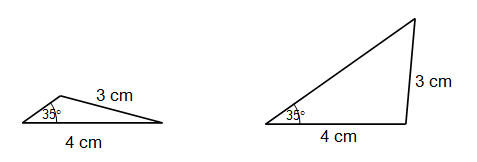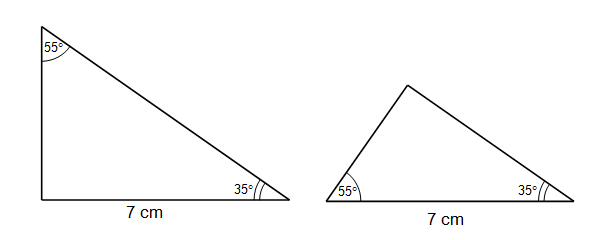Home > Geometric reasoning > Good teaching > Exploring congruence > Developing the congruence tests

# Developing the congruence tests

With congruent triangles, many students do not understand the importance of the qualifiers of 'included angle' in the SAS test nor the 'corresponding side' in the AAS test. Further explanation of the tests can be found in Congruence.

To convince students of the validity of the congruence tests for triangles, investigate all the triangles which satisfy three given conditions. By constructing the triangles, both in a pen and paper environment and in a virtual environment, students realise that there can be more than one triangle constructed.

For example, these two triangles both have an angle of 35° and sides of 3 cm and 4 cm but they are not congruent.In the same way, simply specifying two angles and a side does not produce a unique triangle. These two triangles both have angles of 35° and 55° and a side of 7 cm but they are not congruent.After engaging in construction activities, students will develop a more meaningful understanding of the tests.

## Paper, pencil and protractor

In this activity, students construct all possible triangles which have three given measurements. They determine the conditions under which congruence can be guaranteed.

## Virtual congruence

In this activity, students manipulate virtual diagrams and confirm the congruence tests. Once the triangles are constructed, they can be transformed and superimposed on each other.